## mht.wtf

A blog about computer science, programming, and whatnot.

# Content Aware Image Resize

February 13, 2017

Content aware image resizing, liquid image resizing, retargeting, or seam carving, refers to a image resizing technique where one can insert or remove seams, or “paths of least importance”, in order to shrink or grow the image. I was introduced to the concept by a YouTube video by Shai Avidan and Ariel Shamir.

In this blog post, I’ll go through a simple proof-of-concept implementation of content aware image resizing, naturally in Rust :)

For our sample image, I simply searched1 for "sample image", and got back this2: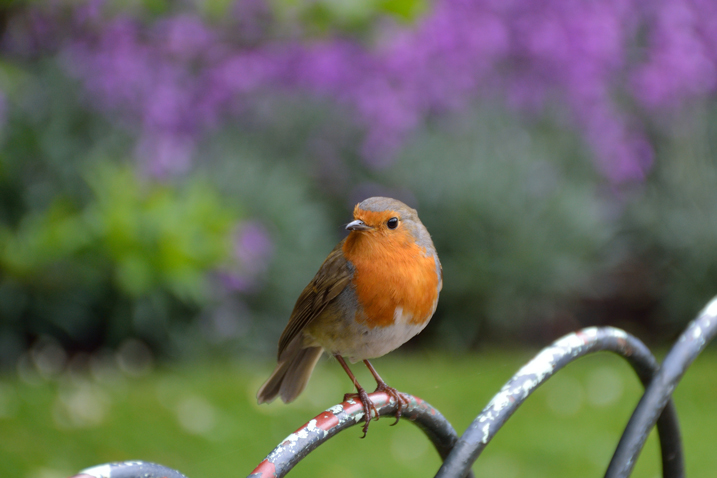# Sketching out a top down approach

Let’s start with some brainstorming. I imagine the library to be used like this:

/// caller.rs
let mut image = car::load_image(path);
// Resize to a known size?
image.resize_to(car::Dimensions::Absolute(800, 580));
// or remove 20 rows?
image.resize_to(car::Dimensions::Relative(0, -20));
// Maybe show the image in a window?
car::show_image(&image);
// or save to disk?
image.save("resized.jpeg");

The most important functions in lib.rs could look something like this:

/// lib.rs
pub fn load_image(path: Path) -> Image {
// We'll forget about error handling for now :)
Image {
}
}

impl Image {
pub fn resize_to(&mut self, dimens: Dimensions) {
// How many columns and rows do we need to insert/remove?
let (mut xs, mut ys) = self.size_diffs(dimens);
// When we want to add columns and rows, we would like
// to always pick the path with the lowest score, no
// matter if it's a row or a column.
while xs != 0 && ys != 0 {
let best_horizontal = image.best_horizontal_path();
let best_vertical = image.best_vertical_path();
// Insert the best
if best_horizontal.score < best_vertical.score {
self.handle_path(best_horizontal, &mut xs);
} else {
self.handle_path(best_vertical, &mut ys);
}
}
// Insert the rest in either direction.
while xs != 0 {
let path = image.best_horizontal_path();
self.handle_path(path, &mut xs);
}
while ys != 0 {
let path = image.best_vertical_path();
self.handle_path(path, &mut ys);
}
}
}

This gives us some idea on how to approach writing system. We need to load an image, we need to find these seams, or paths, and we need to handle removing such a path from the image. In addition, we would perhaps like to be able to see our result.

Let’s do the image loading first, so we know what kind of API we’re working with.

## image

The image library from the Piston developers seems useful, so we’ll add image = "0.12" to our Cargo.toml. A quick search in the docs is all that it takes for us to write the image loading:

struct Image {
inner: image::DynamicImage,
}

impl Image {
pub fn load_image(path: &Path) -> Image {
Image {
inner: image::open(path).unwrap()
}
}
}

A natural next step is figuring out how to get the gradient magnitudes from a image::DynamicImage. The image crate doesn’t provide a way to do this directly, but the imageproc crate does: imageproc::gradients::sobel_gradients. Here however, we run into trouble3. The sobel_gradient function takes an 8-bit grayscale image, and returns a 16-bit grayscale image. The image we have loaded is an RGB image with 8-bits per channel, so we’ll have to decompose the channels, convert the three channels into separate grayscale images, compute the gradients of the three component images, and then merge the gradients together into one image, in which we will do the path searching.

Is this elegant? No. Does it work? Maybe :)

type GradientBuffer = image::ImageBuffer<image::Luma<u16>, Vec<u16>>;

impl Image {
pub fn load_image(path: &Path) -> Image {
Image {
inner: image::open(path).unwrap()
}
}

// We'll assume RGB
let (red, green, blue) = decompose(&self.inner);

let (w, h) = r_grad.dimensions();
let mut container = Vec::with_capacity((w * h) as usize);
container.push(r + g + b);
}
image::ImageBuffer::from_raw(w, h, container).unwrap()
}
}

fn decompose(image: &image::DynamicImage) -> (image::DynamicImage,
image::DynamicImage,
image::DynamicImage) {
let w = image.width();
let h = image.height();
let mut red = image::DynamicImage::new_luma8(w, h);
let mut green = image::DynamicImage::new_luma8(w, h);
let mut blue = image::DynamicImage::new_luma8(w, h);
for (x, y, pixel) in image.pixels() {
let r = pixel;
let g = pixel;
let b = pixel;
red.put_pixel(x, y, *image::Rgba::from_slice(&[r, r, r, 255]));
green.put_pixel(x, y, *image::Rgba::from_slice(&[g, g, g, 255]));
blue.put_pixel(x, y, *image::Rgba::from_slice(&[b, b, b, 255]));
}
(red, green, blue)
}

When ran, Image::gradient_magnitune takes our bird image, and returns this: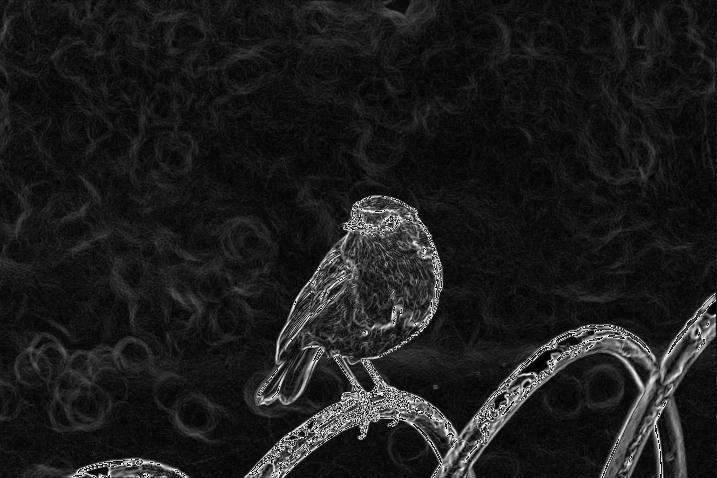## The path of least resistance

Now we have to implement the arguably hardest part of the program: the DP algorithm to find the path of least resistance. Let’s take a quick look at how this will work out. For simplicitys sake, we’ll only look at the case where we find a vertical path. Imagine the table below being the gradient image of a 6x6 image.

$$G = \begin{bmatrix} 1 & 4 & 3 & 4 & 2 & 1\\\ 2 & 2 & 3 & 5 & 3 & 2\\\ 1 & 4 & 5 & 5 & 1 & 2\\\ 4 & 4 & 3 & 1 & 5 & 3\\\ 5 & 3 & 2 & 2 & 3 & 1\\\ 3 & 1 & 4 & 4 & 1 & 1 \end{bmatrix}$$

The point of the algorithm is to find a path $P=p_1 \dots\ p_6$ from one of the top cells $G_{1i}$ to one of the bottom cells $G_{6j}$, such that we minimize $\sum_{1 \leq i \leq 6} p_i$. This can be done by creating a new table $S$ using the following recurrence relation (ignoring boundaries):

$$S_{ji} = \begin{cases} G_{6i} & \text{ if } i = 6\\ G_{ji} + \min(S_{j + 1, i - 1}, S_{j + 1, i}, S_{j + 1, i + 1}) & \text{ otherwise} \end{cases}$$

That is, each cell in $S$ is the minimum sum from that cell to a cell on the bottom. Every cell selects the smallest of the three cells below it in the table to be the next cell in the path. When we have completed $S$, we simply select the smallest number in the top row to be our start.

Let’s find $S$:

$$S^{(1)} = \begin{bmatrix} - & - & - & - & - & -\\\ - & - & - & - & - & -\\\ - & - & - & - & - & -\\\ - & - & - & - & - & -\\\ - & - & - & - & - & -\\\ 3 & 1 & 4 & 4 & 1 & 1 \end{bmatrix} \hspace{1cm} S^{(2)} = \begin{bmatrix} - & - & - & - & - & -\\\ - & - & - & - & - & -\\\ - & - & - & - & - & -\\\ - & - & - & - & - & -\\\ 6 & 4 & 3 & 3 & 4 & 2\\\ 3 & 1 & 4 & 4 & 1 & 1 \end{bmatrix}$$ $$S^{(3)} = \begin{bmatrix} - & - & - & - & - & -\\\ - & - & - & - & - & -\\\ - & - & - & - & - & -\\\ 8 & 7 & 6 & 4 & 7 & 5\\\ 6 & 4 & 3 & 3 & 4 & 2\\\ 3 & 1 & 4 & 4 & 1 & 1 \end{bmatrix} \hspace{1cm} S^{(4)} = \begin{bmatrix} - & - & - & - & - & -\\\ - & - & - & - & - & -\\\ 8 & 10 & 9 & 9 & 5 & 7\\\ 8 & 7 & 6 & 4 & 7 & 5\\\ 6 & 4 & 3 & 3 & 4 & 2\\\ 3 & 1 & 4 & 4 & 1 & 1 \end{bmatrix}$$ $$S^{(5)} = \begin{bmatrix} \ - & - & - & - & - & -\\\ 10 & 10 & 12 & 10 & 8 & 7\\\ 8 & 10 & 9 & 9 & 5 & 7\\\ 8 & 7 & 6 & 4 & 7 & 5\\\ 6 & 4 & 3 & 3 & 4 & 2\\\ 3 & 1 & 4 & 4 & 1 & 1 \end{bmatrix} \hspace{1cm} S^{(6)} = \begin{bmatrix} 11 & 14 & 13 & 13 & 10 & \textbf{8}\\\ 10 & 10 & 12 & 10 & 8 & \textbf{7}\\\ 8 & 10 & 9 & 9 & \textbf{5} & 7\\\ 8 & 7 & 6 & \textbf{4} & 7 & 5\\\ 6 & 4 & 3 & \textbf{3} & 4 & 2\\\ 3 & 1 & 4 & 4 & \textbf{1} & 1 \end{bmatrix}$$

And there it is! We can see that there is a path which sums to only 8, and that the path starts in the upper right corner. In order to find the path, we could have saved which way we went for each cell (left, down, or right), but we don’t have to: we can simply choose the minimum child of each cell, because the cells in $S$ says how long the shortest path from that cell to a bottom cell is.

Also note that there are two paths that sum to 8 (the two bottom cells differ in the two paths).

### Implementation

Since we are just prototyping we will do the simplest thing. We’ll make a struct with an array for the table, and just for loop our way through the algorithm.

struct DPTable {
width: usize,
height: usize,
table: Vec<u16>,
}

impl DPTable {
let dims = gradient.dimensions();
let w = dims.0 as usize;
let h = dims.1 as usize;
let mut table = DPTable {
width: w,
height: h,
table: vec![0; w * h],
};
// return gradient[h][w], save us some typing
let get = |w, h| gradient.get_pixel(w as u32, h as u32);

// Initialize bottom row
for i in 0..w {
let px = get(i, h - 1);
table.set(i, h - 1, px)
}
// For each cell in row j, select the smaller of the cells in the
// row above. Special case the end rows
for row in (0..h - 1).rev() {
for col in 1..w - 1 {
let l = table.get(col - 1, row + 1);
let m = table.get(col    , row + 1);
let r = table.get(col + 1, row + 1);
table.set(col, row, get(col, row) + min(min(l, m), r));
}
// special case far left and far right:
let left = get(0, row) + min(table.get(0, row + 1), table.get(1, row + 1));
table.set(0, row, left);
let right = get(0, row) + min(table.get(w - 1, row + 1), table.get(w - 2, row + 1));
table.set(w - 1, row, right);
}
table
}
}

After running, we can convert the DPTable back to a GradientBuffer, and write it to a file. The pixels in the image below are the path weights divided by 128.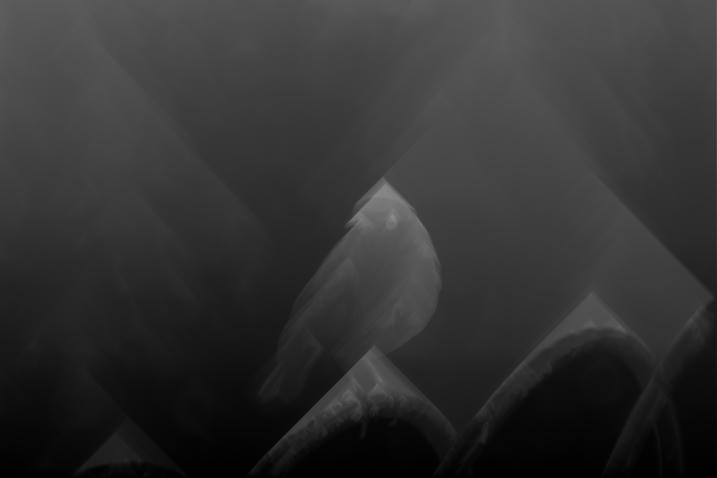The image can be interpreted as follows: white pixels are cells that have a large sum from it to the bottom. These pixels has so much detail (change of color) around it (which we would like to preserve) so the gradient, which tells something about the rate of change, is large. Since the path finding algorithm will search for the smallest sum, which here is the “darkest path”, the algorithm will try its best to avoid these pixels. That is, the white parts in the gradient image are the most distinct parts.

## Finding the path

Now that we have the entire table, finding the best path is easy: it’s just a matter of searching through the uppper row and creating a vec of indices, by always choosing the smallest child:

impl DPTable {
fn path_start_index(&self) -> usize {
// Has FP Gone Too Far?!
self.table.iter()
.take(self.width)
.enumerate()
.map(|(i, n)| (n, i))
.min()
.map(|(_, i)| i)
.unwrap()
}
}

struct Path {
indices: Vec<usize>,
}

impl Path {
pub fn from_dp_table(table: &DPTable) -> Self {
let mut v = Vec::with_capacity(table.height);
let mut col: usize = table.path_start_index();
v.push(col);
for row in 1..table.height {
// Leftmost, no child to the left
if col == 0 {
let m = table.get(col, row);
let r = table.get(col + 1, row);
if m > r {
col += 1;
}
// Rightmost, no child to the right
} else if col == table.width - 1 {
let l = table.get(col - 1, row);
let m = table.get(col, row);
if l < m {
col -= 1;
}
} else {
let l = table.get(col - 1, row);
let m = table.get(col, row);
let r = table.get(col + 1, row);
let minimum = min(min(l, m), r);
if minimum == l {
col -= 1;
} else if minimum == r {
col += 1;
}
}
v.push(col + row * table.width);
}

Path {
indices: v
}
}
}

In order to see if the paths selected are at least plausible, I generated 10 paths, and colored them yellow: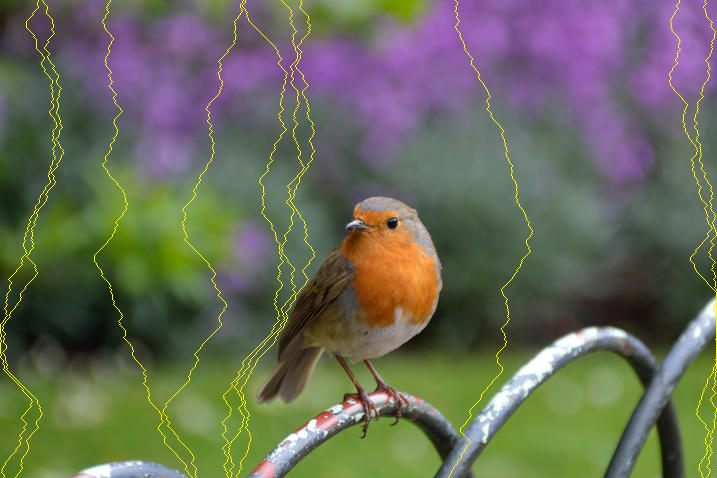Looks plausible to me!

## Removal

The only thing remaining now is to remove the path instead of coloring it yellow. Since we simply want to get something to work, we could do this in a pretty simple way: get the raw bytes from the image, and copy the intervals between in indexes we want to remove over in a new array, which we create a new image from.

impl Image {
fn remove_path(&mut self, path: Path) {
let image_buffer = self.inner.to_rgb();
let (w, h) = image_buffer.dimensions();
let container = image_buffer.into_raw();
let mut new_pixels = vec![];

let mut path = path.indices.iter();
let mut i = 0;
while let Some(&index) = path.next() {
new_pixels.extend(&container[i..index * 3]);
i = (index + 1) * 3;
}
new_pixels.extend(&container[i..]);
let ib = image::ImageBuffer::from_raw(w - 1, h, new_pixels).unwrap();
self.inner = image::DynamicImage::ImageRgb8(ib);
}
}

Finaly, the time has come. Now we can remove a line from an image, or we could loop, and remove, say, 200 lines:

let mut image = Image::load_image(path::Path::new("sample-image.jpg"));
for _ in 0..200 {
let path = Path::from_dp_table(&table);
image.remove_path(path);
}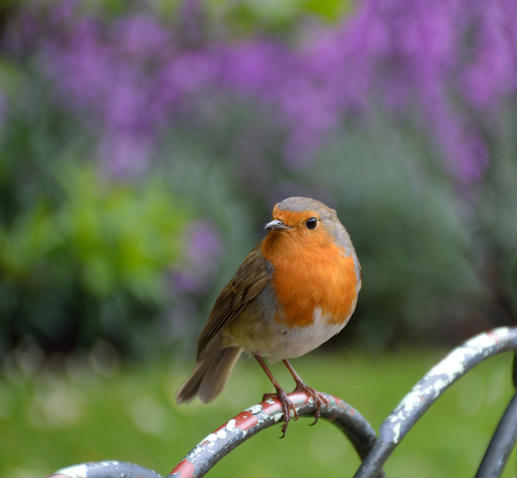However, we can see that the algorithm has removed quite a lot of the right side of the image, that is, the image is more or less cropped, which was exactly one of the problems that we would like to solve! A quick and somewhat dirty fix to this is to simply alter the gradient a little, by explicitly setting the borders to some large number, say 100.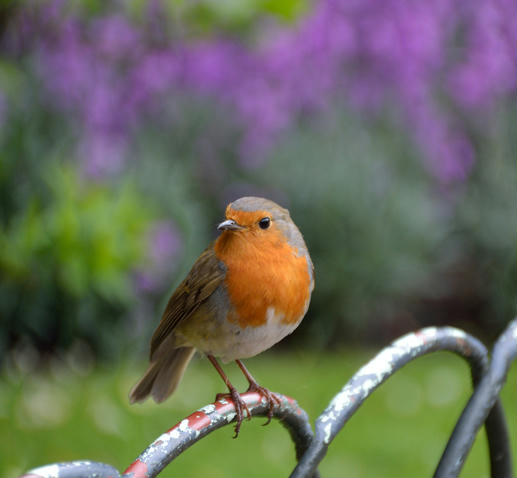There are quite a few artifacts here, which makes the end result a little less satisfactory. The bird however is almost untouched, and still looks great (to me). You could also argue that we have destroyed all sense of image composition in the process of making this image only slightly smaller. To this I will say …. uum…. yes.

## Seeing is believing

Saving the images to a file and looking at it is kind of cool, but it isn’t resize-window-live-update cool! As a final effort, let’s try to hack something together.

First, we need to be able to load, get, and resize an image outside of the crate. We’ll try to make something like our initial plan:

extern crate content_aware_resize;
use content_aware_resize as car;

fn main() {
let mut image = car::load_image(path);
image.resize_to(car::Dimensions::Relative(-1, 0));
let data: &[u8] = image.get_image_data();
// Somehow show this data in a window
}

We start simple, by only adding exactly what we need, and taking shortcuts where we can.

pub enum Dimensions {
Relative(isize, isize),
}
...
impl Image {
fn size_difference(&self, dims: Dimensions) -> (isize, isize) {
let (w, h) = self.inner.dimensions();
match dims {
Dimensions::Relative(x, y) => {
(w as isize + x, h as isize + x)
}
}
}

pub fn resize_to(&mut self, dimensions: Dimensions) {
let (mut xs, mut _ys) = self.size_difference(dimensions);
// Only horizontal downsize for now
if xs < 0 { panic!("Only downsizing is supported.") }
if _ys != 0 { panic!("Only horizontal resizing is supported.") }
while xs > 0 {
let path = Path::from_dp_table(&table);
self.remove_path(path);
xs -= 1;
}
}

pub fn get_image_data(&self) -> &[u8] {
self.inner.as_rgb8().unwrap()
}
}

Just a little copy-paste!

Now, maybe we want the resizable window. We can start a new project, include the library crate, and use, say, sdl2 to get something up fast.

extern crate content_aware_resize;
extern crate sdl2;
use content_aware_resize as car;
use sdl2::rect::Rect;
use sdl2::event::{Event, WindowEvent};
use sdl2::keyboard::Keycode;
use std::path::Path;

fn main() {
let mut image = car::Image::load_image(Path::new("sample-image.jpeg"));
let (mut w, h) = image.dimmensions();

// Setup sdl2 stuff, and get a window
let sdl_ctx = sdl2::init().unwrap();
let video = sdl_ctx.video().unwrap();
let window = video.window("Context Aware Resize", w, h)
.position_centered()
.opengl()
.resizable()
.build()
.unwrap();

let mut renderer = window.renderer().build().unwrap();

// Convenience function to update texture with a resized image
let update_texture = |renderer: &mut sdl2::render::Renderer, image: &car::Image| {
let (w, h) = image.dimmensions();
let pixel_format = sdl2::pixels::PixelFormatEnum::RGB24;
let mut tex = renderer.create_texture_static(pixel_format, w, h).unwrap();
let data = image.get_image_data();
let pitch = w * 3;
tex.update(None, data, pitch as usize).unwrap();
tex
};
let mut texture = update_texture(&mut renderer, &image);

let mut event_pump = sdl_ctx.event_pump().unwrap();
'running: loop {
for event in event_pump.poll_iter() {
// Handle exit and resize events
match event {
Event::Quit {..}
| Event::KeyDown { keycode: Some(Keycode::Escape), .. } => { break 'running },
Event::Window {win_event: WindowEvent::Resized(new_w, _h), .. } => {
// Find out how many pixels we sized down, and scale down
// the image accordingly
let x_diff = new_w as isize - w as isize;
if x_diff < 0 {
image.resize_to(car::Dimensions::Relative(x_diff, 0));
}
w = new_w as u32;
texture = update_texture(&mut renderer, &image);
},
_ => {}
}
}
// Clear, copy, and present.
renderer.clear();
renderer.copy(&texture, None, Some(Rect::new(0, 0, w, h))).unwrap();
renderer.present();
}
}

And that’s it. A days work, wih only very little knowledge of sdl2, image, and blog post writing. I hope you enjoyed it, if only just a little bit :)

1. Somehow, duckduckgoed doesn’t work as well as googled when used as a verb. ↩︎

2. I’d like to know if there is an easier way to do this! In addition, saving the resulting gradient is seemingly not possible at the moment, as the function returns an ImageBuffer over u16, while ImageBuffer::save requires the underlying data to be u8. I also couldn’t figure out how to create a DynamicImage (which also has a ::save, with a slightly cleaner interface) from an ImageBuffer, but this might be possible. ↩︎